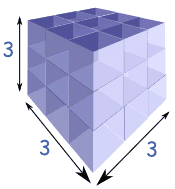Definition of

# Cube NumberThe result of multiplying a whole number by itself twice.

Example: 3 × 3 × 3 = 27, so 27 is a cube number.

The whole number is used three times, just like the sides of a cube.

Here are the first few cube numbers:
1 (=1×1×1)
8 (=2×2×2)
27 (=3×3×3)
64 (=4×4×4)
125 (=5×5×5)
... etc